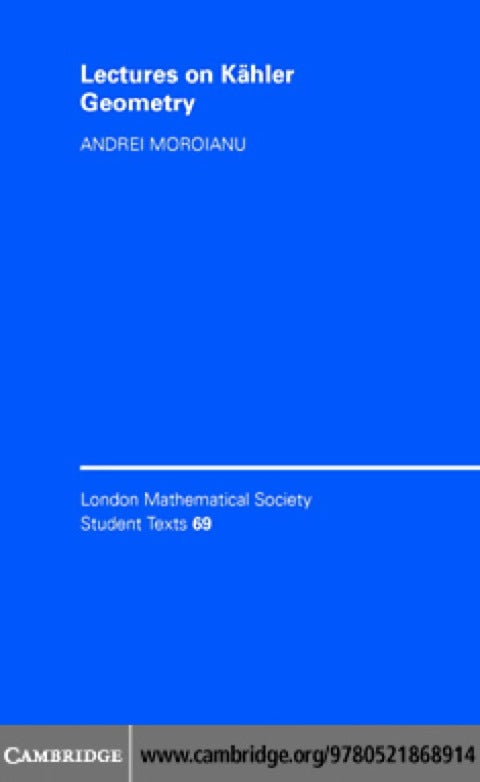• Author(s) Andrei Moroianu
• Edition
• Published03292007
• PublisherCambridge University Press
• ISBN9780511271694
Kähler geometry is a beautiful and intriguing area of mathematics, of substantial research interest to both mathematicians and physicists. This self-contained graduate text provides a concise and accessible introduction to the topic. The book begins with a review of basic differential geometry, before moving on to a description of complex manifolds and holomorphic vector bundles. Kähler manifolds are discussed from the point of view of Riemannian geometry, and Hodge and Dolbeault theories are outlined, together with a simple proof of the famous Kähler identities. The final part of the text studies several aspects of compact Kähler manifolds: the Calabi conjecture, Weitzenböck techniques, Calabi–Yau manifolds, and divisors. All sections of the book end with a series of exercises and students and researchers working in the fields of algebraic and differential geometry and theoretical physics will find that the book provides them with a sound understanding of this theory.

# Lectures on Kähler Geometry

##### Get it instantly

Zookal account needed

\$54.36 \$57.20 Save \$2.84
• Author(s) Andrei Moroianu
• Edition
• Published03292007
• PublisherCambridge University Press
• ISBN9780511271694
Kähler geometry is a beautiful and intriguing area of mathematics, of substantial research interest to both mathematicians and physicists. This self-contained graduate text provides a concise and accessible introduction to the topic. The book begins with a review of basic differential geometry, before moving on to a description of complex manifolds and holomorphic vector bundles. Kähler manifolds are discussed from the point of view of Riemannian geometry, and Hodge and Dolbeault theories are outlined, together with a simple proof of the famous Kähler identities. The final part of the text studies several aspects of compact Kähler manifolds: the Calabi conjecture, Weitzenböck techniques, Calabi–Yau manifolds, and divisors. All sections of the book end with a series of exercises and students and researchers working in the fields of algebraic and differential geometry and theoretical physics will find that the book provides them with a sound understanding of this theory.